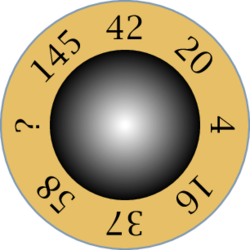Fichier enigme01.mp (figure 1) — Modifié le 15 Mars 2010 à 08 h 56

## Quel est le nombre absent?Source


verbatimtex
%&latex
\documentclass{minimal}
\usepackage{lucimatx}
\begin{document}
etex

beginfig(1);

fill fullcircle scaled 76 withcolor (0.9,0.75,0.4);
draw fullcircle scaled 76 withcolor (0.5,0.6,0.7);

label(btex $4$ etex rotated -90, (30,0));
label(btex $20$ etex rotated -45, (30/sqrt(2),30/sqrt(2)));
label(btex $42$ etex , (0,30));
label(btex $145$ etex rotated 45, (-30/sqrt(2),30/sqrt(2)));
label(btex ? etex rotated 90, (-30,0));
label(btex $58$ etex rotated 135, (-30/sqrt(2),-30/sqrt(2)));
label(btex $37$ etex rotated 180, (0,-30));
label(btex $16$ etex rotated -135, (30/sqrt(2),-30/sqrt(2)));

for i:=1 upto 46:
fill fullcircle scaled (47-i) withcolor (i/45)*white;
endfor;

endfig;

end